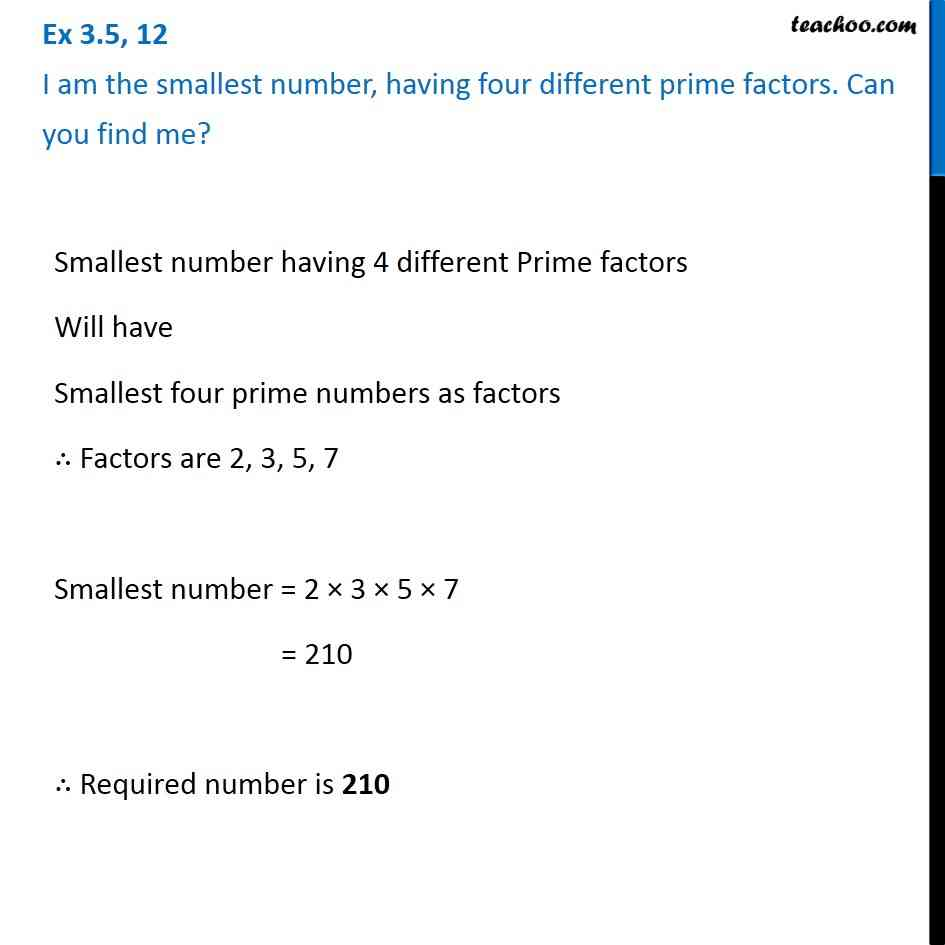1. Chapter 3 Class 6 Playing with Numbers
2. Concept wise
3. Prime Factorisation

Transcript

Ex 3.5, 12 I am the smallest number, having four different prime factors. Can you find me?Smallest number having 4 different Prime factors Will have Smallest four prime numbers as factors ∴ Factors are 2, 3, 5, 7 Smallest number = 2 × 3 × 5 × 7 = 210 ∴ Required number is 210

Prime Factorisation